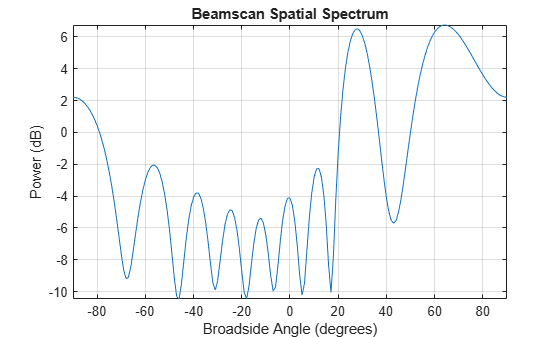# Beamscan Direction-of-Arrival Estimation

This example shows how to use the nonparametric beamscan technique to estimate the directions of arrival (DOA) of two signals. The beamscan algorithm estimates the DOAs by scanning the array beam over a region of interest. The algorithm computes the output power for each beamscan angle and identifies the maxima as the DOA estimates.

Note: This example runs only in R2016b or later. If you are using an earlier release, replace each call to the function with the equivalent `step` syntax. For example, replace `myObject(x)` with `step(myObject,x)`.

Construct a uniform linear array (ULA) consisting of ten isotropic antenna elements. The carrier frequency of the incoming narrowband sources is 1 GHz.

```fc = 1e9; lambda = physconst('LightSpeed')/fc; antenna = phased.IsotropicAntennaElement('FrequencyRange',[8e8 1.2e9]); array = phased.ULA('Element',antenna,'NumElements',10,'ElementSpacing',lambda/2);```

The incident wavefield consists of linear FM pulses from two sources. The DOAs of the two sources are 30° azimuth and 60° azimuth. Both sources have elevation angles of 0°.

```waveform = phased.LinearFMWaveform('SweepBandwidth',1e5,... 'PulseWidth',5e-6,'OutputFormat','Pulses','NumPulses',1); sig1 = waveform(); sig2 = sig1; ang1 = [30; 0]; ang2 = [60;0]; arraysig = collectPlaneWave(array,[sig1 sig2],[ang1 ang2],fc); rng default npower = 0.01; noise = sqrt(npower/2)*... (randn(size(arraysig)) + 1i*randn(size(arraysig))); rxsig = arraysig + noise;```

Implement a beamscan DOA estimator. Scan the azimuth angles from $-90°$ to $90°$. Output the DOA estimates, and plot the spatial spectrum. The locations of the two largest peaks of the spectrum identify the DOAs of the signals.

```estimator = phased.BeamscanEstimator('SensorArray',array,... 'OperatingFrequency',fc,'ScanAngles',-90:90,... 'DOAOutputPort',true,'NumSignals',2); [y,sigang] = estimator(rxsig); disp(sigang)```
``` 64 28 ```

Plot the spatial spectrum as a function of broadside angle.

`plotSpectrum(estimator)`